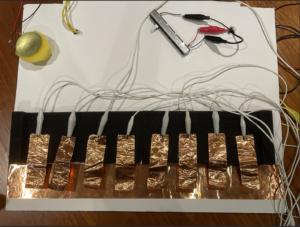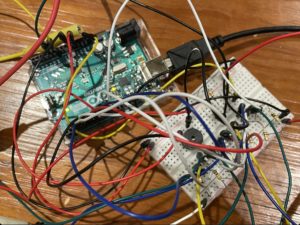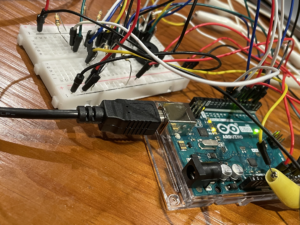# Jade and Bhavicka’s Keys of Misery

Description / MechanismFor this week, Bhavicka and I made a keyboard with a little drum around. We planned to make something like a DJ mixer, so we used the copper tapes as a keyboard, piezo as a drum and the slider as the sliding control.

At the very beginning, we thought about using MP3 shield, AD card and another speaker to play MP3 files of the sound for drum and keyboard. But it was too complicated because of all the codes that are beyond our understanding, libraries that should be installed and some compatible issues, so eventually it didn’t work out.

Then there is plan B. We used four notes for the drum sound, making it sounds like an opening theme. For the keys, we used C, D, E, F, G, A, B, C (higher) in order. The slider could change the pitch of the keys, so the pitch gets higher as we slide it to the middle and even higher when it reaches the other end.

Code

```# include "pitches.h"

const int knockSensor = A0;
const int buzzer = 7;
const int slide = A1;
const int threshold = 1000;

const int note1 = 2;
const int note2 = 3;
const int note3 = 4;
const int note4 = 5;
const int note5 = 6;
const int note6 = 8;
const int note7 = 9;
const int note8 = 10;

int drum = {NOTE_C1, NOTE_C2, NOTE_C3, NOTE_C4};

int pitch0 = {NOTE_C3, NOTE_D3, NOTE_E3, NOTE_F3, NOTE_G3, NOTE_A4, NOTE_B4, NOTE_C4};
int pitch1 = {NOTE_C4, NOTE_D4, NOTE_E4, NOTE_F4, NOTE_G4, NOTE_A5, NOTE_B5, NOTE_C5};
int pitch2 = {NOTE_C5, NOTE_D5, NOTE_E5, NOTE_F5, NOTE_G5, NOTE_A6, NOTE_B6, NOTE_C6};

int pitch = 0;

void setup() {
Serial.begin(9600);
pinMode(note1, INPUT);
pinMode(note2, INPUT);
pinMode(note3, INPUT);
pinMode(note4, INPUT);
pinMode(note5, INPUT);
pinMode(note6, INPUT);
pinMode(note7, INPUT);
pinMode(note8, INPUT);
}

void loop() {

Serial.print("Knob Value: ");
Serial.print("Slide Value: ");

tone(buzzer, drum, 200);
delay(200);
tone(buzzer, drum, 200);
delay(200);
tone(buzzer, drum, 200);
delay(200);
tone(buzzer, drum, 200);
}

pitch = 0;
}

else if (slideReading <= 880) {
pitch = 1;
}

else {
pitch = 2;
}

if (pitch == 0) {
tone(buzzer, pitch0, 500);

} else if (digitalRead(note2) == HIGH) {
tone(buzzer, pitch0, 500);

}
else if (digitalRead(note3) == HIGH) {
tone(buzzer, pitch0, 500);

}
else if (digitalRead(note4) == HIGH) {
tone(buzzer, pitch0, 500);

}

else if (digitalRead(note5) == HIGH) {
tone(buzzer, pitch0, 500);

}

else if (digitalRead(note6) == HIGH) {
tone(buzzer, pitch0, 500);

}
else if (digitalRead(note7) == HIGH) {
tone(buzzer, pitch0, 500);

}

else if (digitalRead(note8) == HIGH) {
tone(buzzer, pitch0, 500);

} else {
noTone(buzzer);
}
}

else if (pitch == 1) {
tone(buzzer, pitch1, 500);

} else if (digitalRead(note2) == HIGH) {
tone(buzzer, pitch1, 500);

}
else if (digitalRead(note3) == HIGH) {
tone(buzzer, pitch1, 500);

}
else if (digitalRead(note4) == HIGH) {
tone(buzzer, pitch1, 500);

}

else if (digitalRead(note5) == HIGH) {
tone(buzzer, pitch1, 500);

}

else if (digitalRead(note6) == HIGH) {
tone(buzzer, pitch1, 500);

}
else if (digitalRead(note7) == HIGH) {
tone(buzzer, pitch1, 500);

}

else if (digitalRead(note8) == HIGH) {
tone(buzzer, pitch1, 500);

} else {
noTone(buzzer);
}
}

else {
tone(buzzer, pitch2, 500);

} else if (digitalRead(note2) == HIGH) {
tone(buzzer, pitch2, 500);

}
else if (digitalRead(note3) == HIGH) {
tone(buzzer, pitch2, 500);

}
else if (digitalRead(note4) == HIGH) {
tone(buzzer, pitch2, 500);

}

else if (digitalRead(note5) == HIGH) {
tone(buzzer, pitch2, 500);

}

else if (digitalRead(note6) == HIGH) {
tone(buzzer, pitch2, 500);

}
else if (digitalRead(note7) == HIGH) {
tone(buzzer, pitch2, 500);

}

else if (digitalRead(note8) == HIGH) {
tone(buzzer, pitch2, 500);

} else {
noTone(buzzer);
}
}
}```

Our Process

This is the most challenging project so far. We spent a lot of time figuring out the circuits as there were 8 keys, a piezo and a slider. One difficulty we encountered which took us so much time was to close the circuits for the 8 keys.We attached a jumper cable to the copper tape below which connects to power. Then we attached other jumper cables to the keys and connected them to various pins respectively, and we also put resistors to the ground to create a switch. So when the keys touched the copper tape below, this should close a circuit.

Our plan didn’t work out well at first, maybe because we put these key circuits near the circuits for the piezo and slider, which may cause a short circuit. After we moved all the cables to the other side of the bread board, the problem was solved. Since there were so many wires, we had to be really careful to not let them collide with each other, causing short circuits.The coding part was not so challenging as the circuit part. The only problem was about the tone() and noTone() function. We realized that we should use else if condition for different notes.

```else if (digitalRead(note7) == HIGH) {
tone(buzzer, pitch0, 500);
} else if (digitalRead(note8) == HIGH) {
tone(buzzer, pitch0, 500);
} else {
noTone(buzzer);
}```

At first, we wrote the codes like:

```if (digitalRead(note7) == HIGH) {
tone(buzzer, pitch0, 500);
} else {
noTone(buzzer);
}

tone(buzzer, pitch0, 500);
} else {
noTone(buzzer);
}
```

which was problematic because when the other keys were not pressed, then noTone() was always executed. As a result, this caused the buzzer to make strange broken noise.

Although the project is demanding, the process of doing it was interesting and pleasant. The teamwork was great and it was really enjoyable working with Bhavicka!